# Physics - Classical Mechanics - Motion of the Center of Mass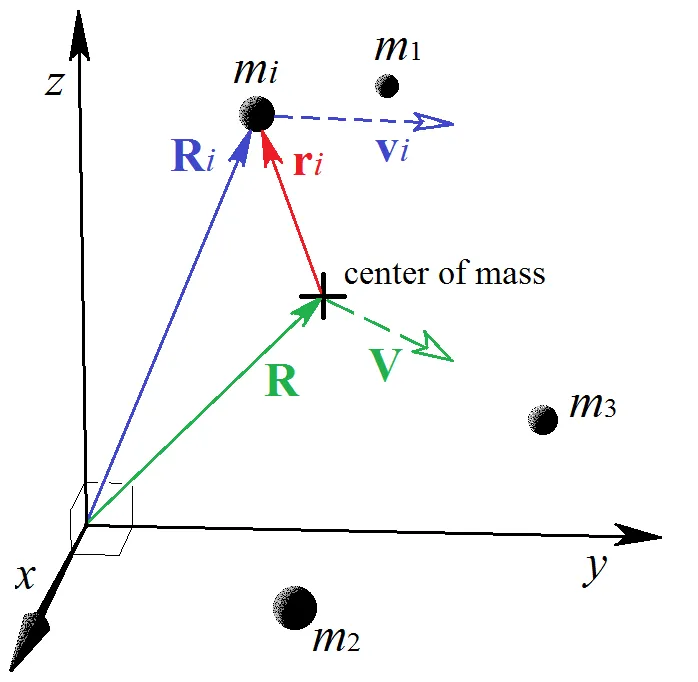[Image 1]

## Introduction

Hey it's a me again @drifter1! Today we continue with Physics and more specifically the branch "Classical Mechanics" to get into the Motion of the Center of Mass. So, without further ado, let's get straight into it!

## Center of Mass

### Definition

The center of mass is defined as a position relative to an object or system of objects. It's the average position of all the weighted, distributed parts of the system, according their masses. Furthermore, it's that unique point in space, where the weighted position vectors of all the parts of a system sum up to zero. For simple rigid objects of uniform density the center of mass is located at the centroid (or geometrical center). For example the center of mass of an equilateral, uniform triangle is at the same position as it's centroid:[ Image 2]

### Usefulness

But, what about other more complicated shapes and objects? We can clearly understand why the mass of any body is distributed around it's center of mass, but how exactly does this imaginary point help us in calculations? Well, applying a force on that exact point, causes only a motion in the direction of motion, without any rotation. This helps us solve mechanics problems with oddly-shaped objects and more complicated systems. For the purposes of calculation, oddly-shaped objects are treated like having all the mass concentrated in a tiny object located at the center of mass. This imaginary object is mostly call the point mass. Pushing a rigid object at its center of mass is equivalent to applying that same push to the point mass. Applying a force to any point other than the center of mass (unbalanced force) causes the object to begin rotating around its center of mass.

### Calculation

Calculating the center of mass is somewhat simple. Talking about systems of objects it can be found by the vector addition of all the weighted position vectors that point to the center of mass of each object in a system. The quickest technique that lets us avoid the use of such vector arithmetic is calculating the center of mass separately for the components along each axis. In two dimensions the center of mass (COM) is calculated such as:So, to generalize for 3 dimensions we can write: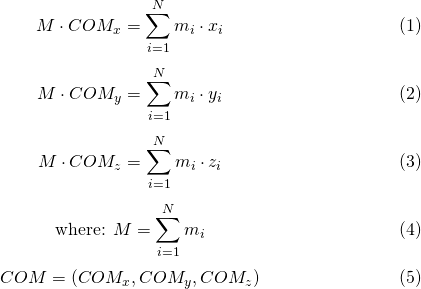The equations 1-3 are for calculating the center of mass for each dimension (x, y, z). Equation 4 is just for simplifying the equations. Equation 5 describes the final point in 3-dimensional space, where the center of mass is at. For continuous distributions of mass, where the sum is not finite, the infinite sum for each axis is expressed in the form of an integral: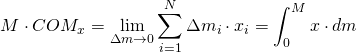Let's now continue with some motion analysis...

## Motion Analysis with Examples

### Velocity of the CM & Total Momentum

Thinking about the equations of before, we understand that we had to use the position (on each axis) of the various particles that each object is build up off to calculate the average point, which is the center of mass. Let's now consider a system of objects/particles with masses m1, m2, m3, ... and distances to the center of mass of r1, r2, r3, ... In terms of the distance from the center of mass from each reference point we can write: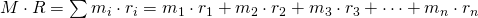Differentiating in respect to time, we of course get the velocity of the center of mass V:Both sides are clearly giving us the total momentum of the system and so we can write the total momentum as: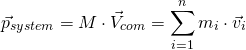Example from Byjus.com:
A boy of mass 25 kg stands on a board of mass 10 kg which in turn is kept on a friction-less horizontal surface. The boy makes a jump with a velocity 5 m/s in the horizontal direction with respect to the board. With what velocity does the board recoil?

Solution:
The initial and final momentum of the system must be conserved and so:So, the board recoils with a velocity of 12.5 m/s in the other direction.

### Acceleration of the CM & Total External Force

Let's now differentiate the previous equation of velocity V again in respect to time. Doing that we of course get the acceleration of the center of mass: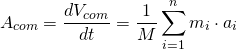Taking the mass to the other side we end up with Newton's second law: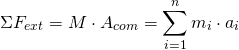The following website has some great illustrative examples:
scripts.mit.edu

## RESOURCES:

### References

2. https://byjus.com/physics/motion-centre-mass/
3. https://scripts.mit.edu/~srayyan/PERwiki/index.php?title=Module_6_--_Center_of_Mass_and_the_motion_of_a_system
4. http://hyperphysics.phy-astr.gsu.edu/hbase/cm.html

### Images

1.2.## Final words | Next up

This is actually it for today's post! Next time we will explain the Physics behind Rocket Propulsion..

See ya!Keep on drifting!

H2
H3
H4
3 columns
2 columns
1 column